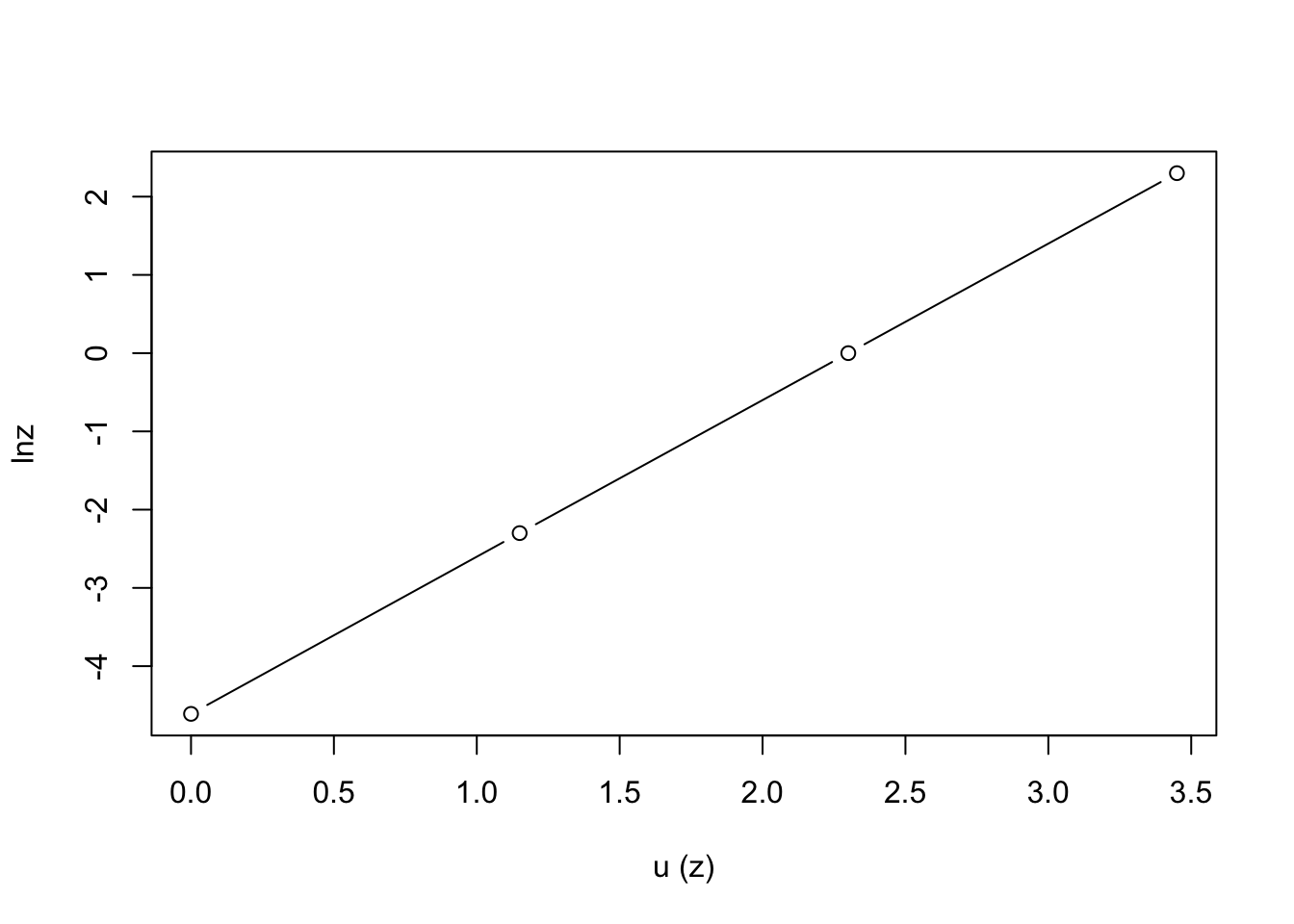## 14.9 Answers to the Problem Set

1. \begin{align*} Re&=\frac{\bar{u}d}{v} \\ &=\frac{(10 m s^{-1})(3m)}{1.5\times10^{-5}m^2s^{-1}}=\frac{30}{1.5\times10^{-5}}=2\times10^6 \end{align*} For $$v=0.1ms^{-1}$$, $$Re=2\times10^4$$

2. Solve the second equation for $$z$$: $z=4x-2$ Substitute this expression into the first equation: $x+2y+3(4x-2)=10$ or $13x+2y=16$ As was probably obvious from the outset, no solution is possible without some further information about either $$x$$, $$y$$ or $$z$$; i.e., we need a third equation. This is an illustration of the so-called closure problem in turbulence which occurs with the Reynolds equations. In simple terms, this problem is “solved” by adding a third empirical equation; one derived from observations, not theory. Thus, if it is observed that usually $$x=-2$$, then we may solve the system of equations: $$y=21$$, $$z=-10$$. Of course, our final answer is in general only as good as the validity of our assumption that $$x=-2$$ for any given case.

Re 3000 6000
$$\bar{u}$$ 1.6 cm s-1 3.2 cm s-1
$$d$$ 0.8 mm 1.0 mm
$$u'/\bar{u}$$ 0.0242 0.0229
$$T$$ 50 ms 33 ms

Two things are of importance to note. First of all, since $$Re$$ is proportional to velocity, and from the problem statement it is fair to assume fluid viscosity, density and flow geometry have stayed the same. It is safe to surmise that $$\overline{u}$$ doubled when $$Re$$ was increased from 3000 to 6000. Secondly, while $$d$$ increased with $$Re$$, which tends to oppose mixing, $$T$$ decreases in larger proportion. In a similar line of argument to that of the text, we may argue that $$T$$ gives an indication of the life span of the average eddy of size $$d$$. Over a time equivalent to several time scales, an eddy can be expected to have become completely mixed with other eddies. Thus, apparently the decrease in $$T$$ more than makes up for the increase in $$d$$, so that mixing becomes more efficient as $$Re$$ gets larger.

1. Equation (14.10) is $$u_*=kz\frac{d\bar u }{dz}$$
rearranging terms $$\frac{k}{u_*}d\bar u =\frac{dz}{z}$$
integrating $$\frac{k}{u_*} \int_0^{\bar u} d \bar u=\int_0^z\frac{dz}{z}$$ $\frac{k\bar u}{u_*}=\ln z-\ln0$ But, $$\ln 0$$ is infinite, so we introduce a small constant called the surface roughness ($$z_0$$), and integrate from $$z=z_0$$ to $$z=z$$. Thus, we are saying that the velocity is zero a small distance ($$z_0$$) above the surface $\frac{k\bar u }{u_*}=\ln z-\ln z_0=\ln\left(\frac{z}{z_0}\right)$
$$z$$ $$\ln z$$ $$\overline u(z)= 1/2 \ln(z/0.01)$$
$$z_0=0.01$$ $$\ln z_0= -4.61$$ 0
0.1 -2.3 1.15
1 0 2.30
10 2.3 3.45
u= c(0,1.15,2.30,3.45)
lnz= c(-4.61,-2.3, 0, 2.3)
plot(u, lnz, type="b", xlab="u (z)")1. \begin{align*} Re&=\frac{ud}{v}=\frac{3ms^{-1}\times0.042m}{151\times10^{-7}m^2s^{-1}}=8.3\times10^3 \\ Nu&=ARe^n=0.6Re^{0.5}=55 \\ F_H&=55\times\frac{26mWm^{-1}°C^{-1}}{0.042m}(30-20)°C \\ &=339000\frac{mW}{m^2}=339\frac{W}{m^2} \end{align*}